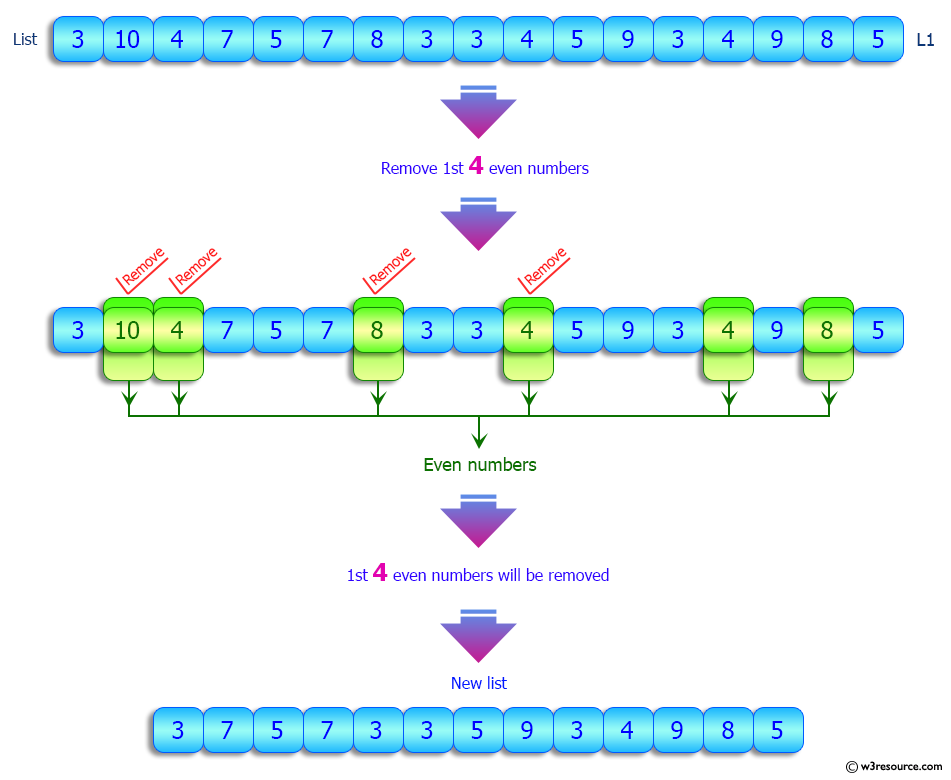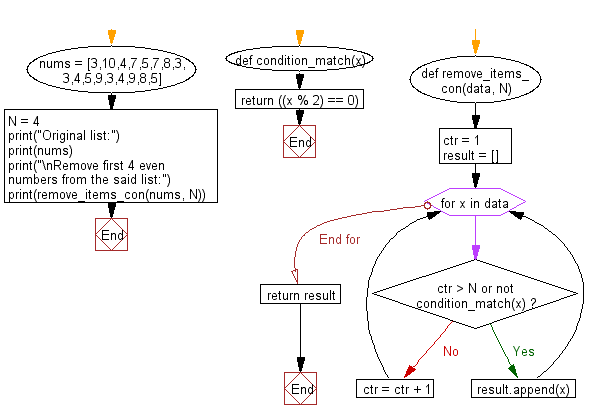﻿ Python: Remove first specified number of elements from a given list satisfying a condition - w3resource# Python: Remove first specified number of elements from a given list satisfying a condition

## Python List: Exercise - 160 with Solution

Write a Python program to remove first specified number of elements from a given list satisfying a condition.

Remove the first 4 number of even numbers from the following list:
[3,10,4,7,5,7,8,3,3,4,5,9,3,4,9,8,5]
Output:
[3, 7, 5, 7, 3, 3, 5, 9, 3, 4, 9, 8, 5]

Sample Solution:

Python Code:

``````def condition_match(x):
return ((x % 2) == 0)
def remove_items_con(data, N):
ctr = 1
result = []
for x in data:
if ctr > N or not condition_match(x):
result.append(x)
else:
ctr = ctr + 1
return result
nums = [3,10,4,7,5,7,8,3,3,4,5,9,3,4,9,8,5]
N = 4
print("Original list:")
print(nums)
print("\nRemove first 4 even numbers from the said list:")
print(remove_items_con(nums, N))
```
```

Sample Output:

```Original list:
[3, 10, 4, 7, 5, 7, 8, 3, 3, 4, 5, 9, 3, 4, 9, 8, 5]

Remove first 4 even numbers from the said list:
[3, 7, 5, 7, 3, 3, 5, 9, 3, 4, 9, 8, 5]
```

Pictorial Presentation:Flowchart:## Visualize Python code execution:

The following tool visualize what the computer is doing step-by-step as it executes the said program:

Python Code Editor:

Have another way to solve this solution? Contribute your code (and comments) through Disqus.

What is the difficulty level of this exercise?

Test your Python skills with w3resource's quiz

﻿

## Python: Tips of the Day

Floor Division:

When we speak of division we normally mean (/) float division operator, this will give a precise result in float format with decimals.

For a rounded integer result there is (//) floor division operator in Python. Floor division will only give integer results that are round numbers.

```print(1000 // 300)
print(1000 / 300)```

Output:

```3
3.3333333333333335```